# Finding Max Flow using the Ford-Fulkerson Algorithm and Matthew McConaughey

## Prereqs

Before reading further, make sure to watch the necessary prerequisite lecture by Matthew McConaughey above. His words of wisdom are the key to all of this.

## What is the Max Flow Problem?

The max flow problem is an optimization problem for determining the maximum amount of stuff that can flow at a given point in time through a single source/sink flow network. A flow network is essentially just a directed graph where the edge weights represent the flow capacity of each edge. The stuff that flows through these networks could be literally anything. Maybe it’s traffic driving through a city, water flowing through pipes, or bits traveling across the internet.

To make this more concrete, let’s look at the following example: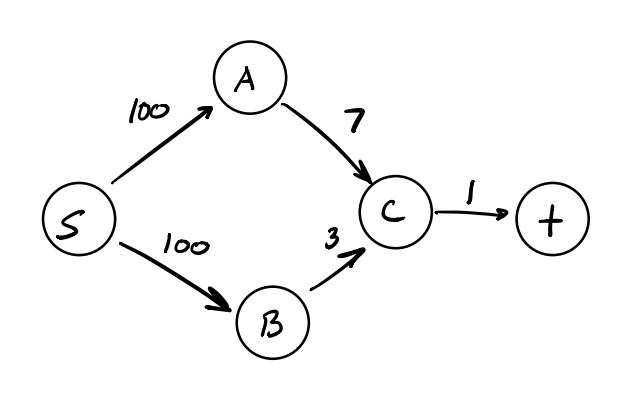This is a pretty simple graph with no back edges where s is the source vertex and t is the sink. Let’s imagine that s is a water treatment facility and t is our home and we’re interested in finding out the amount of water that can flow through to our literal bathroom sink.

You can kind of eyeball this one and see that although the edges coming out of the source (vertex s) have a large capacity, we’re bottlenecked by the edge leading to our home (the sink vertex t) which can only transport 1 unit of water.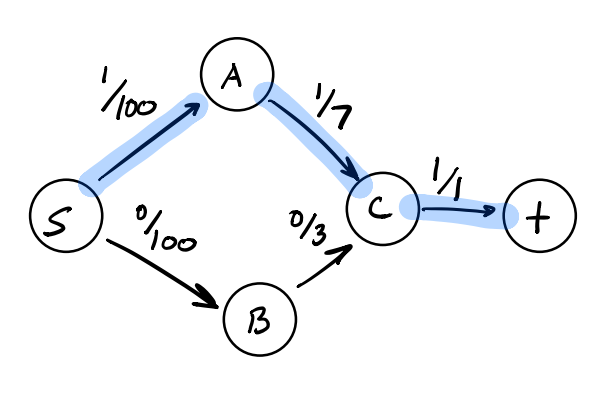Here our flow can clearly be at most the capacity of our smallest edge leading into t. So can we simply look for the smallest capacity edges and say definitively that we know our maximum flow? Almost… and we’ll get to that later with the max-flow min-cut theorem, but first let’s look at a more difficult example that has multiple edges flowing into the sink.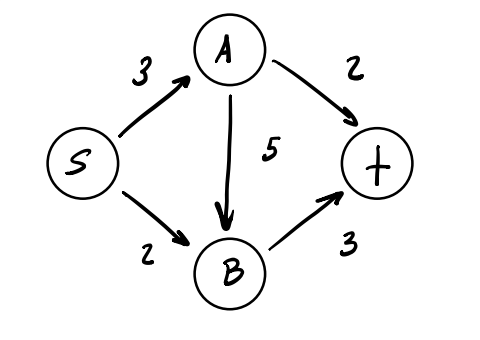The flow network above is a bit of a classic example of this problem. We have four vertices and three paths from s to t. Let’s imagine we have the following greedy algorithm to discover the max flow for this graph:

1. Initially set the flow along every edge to 0.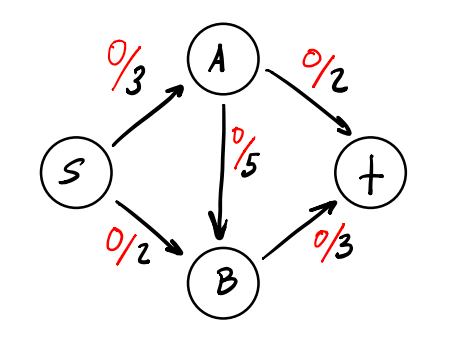1. Use a pathfinding algorithm like depth-first search (DFS) or breadth-first search (BFS) to find a path P from s to t that has available capacity.
2. Let cap(P) indicate the maximum amount of stuff that can flow along this path. To find the capacity of this path, we need to look at all edges e on the path and subtract their current flow, fe, from their capacity ce. We’ll set cap(P) to be equal to the smallest value of ce - fe since this will bottleneck the path.
3. We then augment the flow across the edges in the path P by our cap(P) value.
4. Repeat the process from step 2 until there are no paths left from s to t that have available capacity.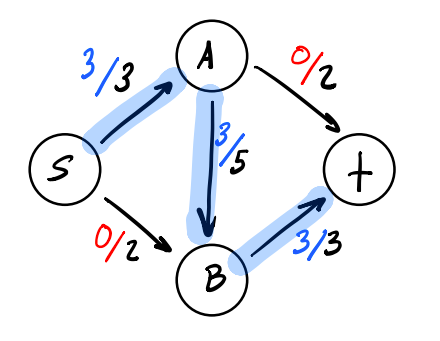Using the naive greedy algorithm described above on our flow network will result in a suboptimal flow of 3. However, with the two edges entering t having capacities of 2 and 3, it really feels like we should be able to achieve a max flow of 5. The graph below shows how we can achieve this by being just a bit smarter with our flow allocations.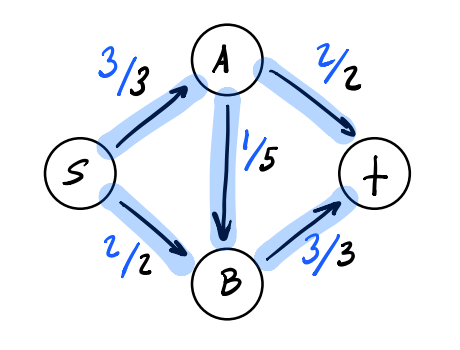How can we do this though? This is where the wisdom of Matthew McConaughey comes in.

“Sometimes you’ve got to go back to actually move forward.”

## The Residual Graph

Imagine if we could look back at the choices we’ve made and undo some of our earlier flow decisions. Turns out we can using something called the residual graph. This is what Matthew was referring to all along.

We’ll basically take our existing graph and update the capacities of all the regular edges to be the current remaining capacity (ce - fe). We’ll then add back edges indicating the amount of flow currently going across this edge. We can then use that back edge to decrease flow in order to try out alternate flow allocations. The graph below shows the residual graph that results after running our original algorithm.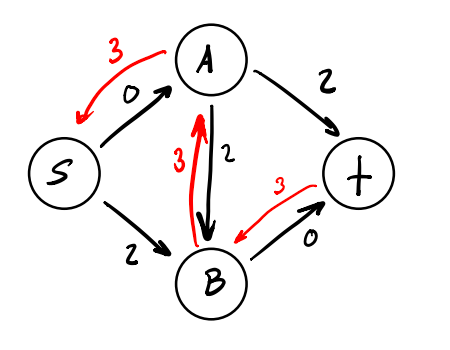With a few tweaks to our algorithm, we can use the concept of a residual graph to find the true maximum flow in our network. This is known as the Ford-Fulkerson algorithm.

## The Ford-Fulkerson Algorithm

This algorithm will look pretty similar to the one we laid out earlier, with one key difference. We will be constructing a residual graph for the flow network and searching for s-t paths across it instead!

1. Initially set the flow along every edge to 0.
2. Construct a residual graph for this network. It should look the same as the input flow network.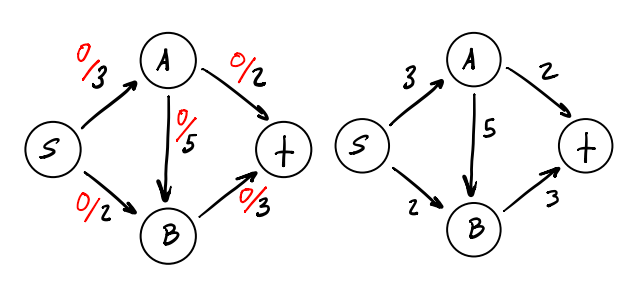1. Use a pathfinding algorithm like depth-first search (DFS) or breadth-first search (BFS) to find a path P from s to t that has available capacity in the residual graph.
2. Let cap(P) indicate the maximum amount of stuff that can flow along this path. To find the capacity of this path, we need to look at all edges e on the path and subtract their current flow, fe, from their capacity ce. We’ll set cap(P) to be equal to the smallest value of ce - fe since this will bottleneck the path.
3. We then augment the flow across the forward edges in the path P by adding cap(P) value. For flow across the back edges in the residual graph, we subtract our cap(P) value.
4. Update the residual graph with these flow adjustments.
5. Repeat the process from step 2 until there are no paths left from s to t in the residual graph that have available capacity.

Let’s step through this for our example graph.

### Step 1

Initial flow is set to 0.### Step 2

Run through the algorithm once and find we can achieve a flow of 3. Update the residual graph.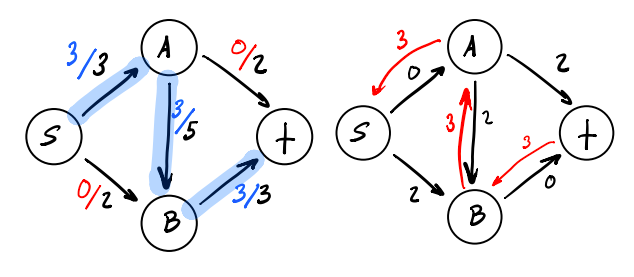### Step 3

Search through the updated residual graph for a new s-t path. There are no forward edges available anymore, but we can use a back edge to augment the current flow. We can decrease the flow along the A-B edge by 2 which will allow us to make use of both edges leading into t!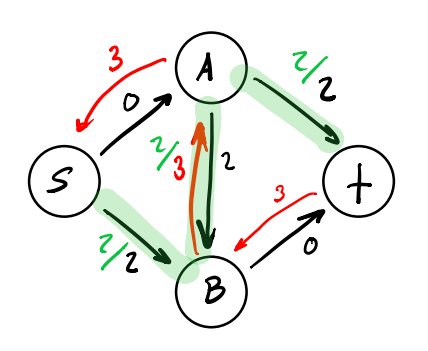### Step 4

Augment the current flow with our findings above and update the residual graph.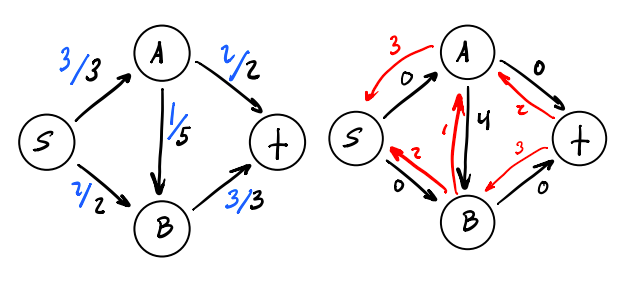There are now no edges with available capacity that we can use to create a path from s to t. This means our run of the Ford-Fulkerson algorithm is complete and our max flow leading into t is 5!

## Summary

That was a pretty trivial example, so I would like to reiterate that the Ford-Fulkerson algorithm can be used to find the max flow of much more complicated flow networks. Provided that they have positive integers as capacities, of course.

I highly recommend watching William Fiset’s video explanation to see an example of the algorithm run against one of these larger networks. Apart from that video, if you’d like to go deeper or just to have it explained in additional ways, I personally found the following lectures, videos, and notes really useful:

Also, if your graph doesn’t have integer capacities or if you want to explore a potentially faster method of finding max flow, I recommend looking into the Edmonds-Karp algorithm.

Best of luck on your algorithmic journey!